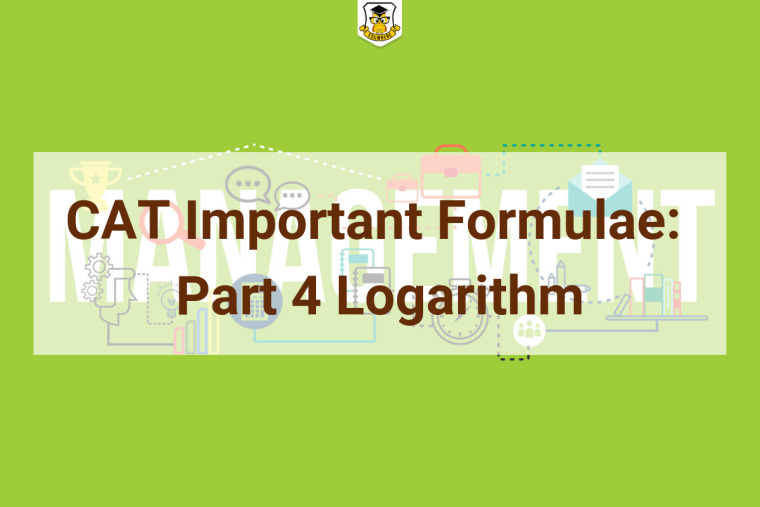## Eduwhere's Concept: LogarithmQuestions on Logarithm have been asked in the CAT exam every year under the quantitative aptitude section. To solve logarithmic questions in CAT, logarithmic formulae are necessary.

Here is a set of important formulae you should know, take a quick look!

1. If n and a are positive real numbers, and a is not equal to 1, then If ax = n, then log a n = x

2. log a n is called logarithmic function. The domain of a logarithmic function is positive real numbers and the range is all real numbers.

3. log of 1 to any base is 0

log b 1 = 0

4. log of any number to base as itself is 1

log a a = 1

5. Logarithm of a Product

log a pq = log a p + loga q

6. Logarithm of a Fraction

log a (p/q) = log a p – log a q

7. log a pn = n log a p

8. a(log a p) = p

9. Base Change Rule of Logarithms

log a n = log b n × log a b

11. if the base is 10, then

log a b = (log b)/(log a)

12. (log a b) × (log b a ) = 1

13. log a b = 1/log b a

14. log (m + n ) is not equal to log m + log n

15. log an p = 1/n (log a p)

16. log an bm = (m/n)log a b

17. log 10 b is called common logarithm because the base is 10

18. log e b is called natural logarithm. It is denoted by ln b.

19. log 1/a b = -log a b

20. log a (1/b) = -log a b

21. log 1/a (1/b) = log a b

1

1198